Statistics materials Back to the Department of Experimental Psychology http://www.psychol.cam.ac.uk/statistics/

This page serves two interrelated purposes. It provides on-line materials in support of statistics taught in the Department of Experimental Psychology in the University of Cambridge, and it provides materials in support of two books:

Within the University of Cambridge, see

Relevant external links to statistics teaching materials and on-line books include:

More general mathematical sites include:

Commercial statistical, mathematical, and graphic software includes

• SPSS -- statistical analysis. Recommended. Originally the name stood for Statistical Program for the Social Sciences.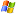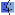• SYSTAT -- statistical analysis.• SigmaStat -- statistical "advisory" software from Systat.• SigmaPlot -- technical graphing software from Systat. Recommended.• S-Plus -- statistical analysis. Note that this is closely related to S, which in turn is closely related to R (see below).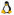• BMDP -- statistical analysis.• SAS -- statistical analysis.• Statistica -- statistical analysis.• GenStat -- statistical analysis.Originally from the Rothamsted agricultural research centre that developed many statistical techniques.
• Mathematica -- symbolic mathematics. Recommended.• Matlab -- numerical and technical computation.• MathType -- equation editing tool.Open-source and otherwise free-to-use software includes

• StatLib archive of information and software.
• The R project. Statistical analysis and graphing.• R operates from a command-line interface (which may be intimidating), but there are also graphical user interfaces (GUIs) for R to make life easier, including The R Commander, SciViews-R, JGR, and RKWard.
• J.J. Faraway's (2002) excellent book Practical Regression and Anova in R (also here), free for personal use, describes how to perform ANOVA and other statistical techniques using R.
• Note that R is closely related to the language S, which is closely related to S-Plus (see above).
• PSPP, an (as yet incomplete) free replacement for SPSS that can read SPSS data/syntax files.• GNUplot. Graphing.• GNU Octave. Numerical and technical computation, with statistical tests, largely compatible with Matlab. The Octave Repository contains more resources.• Maxima, for symbolic mathematics.• OpenOffice contains a spreadsheet program (Calc) that contains some statistical functions.

Power calculation:

Key:Windows,Macintosh,UNIX/Linux;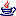Javascript for web browsers;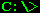MS-DOS or Windows command line.